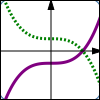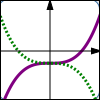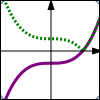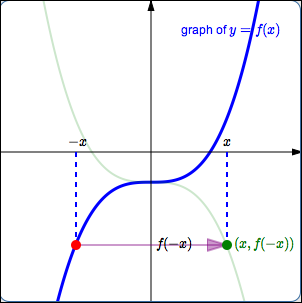﻿ Reflecting About Axes, and the Absolute Value Transformation

# REFLECTING ABOUT AXES, AND THE ABSOLUTE VALUE TRANSFORMATION

• PRACTICE (online exercises and printable worksheets)$y = f(x)$ $y = -f(x)\,$ reflect about the $\,x\,$-axis $y = f(x)$ $y = f(-x)\,$ reflect about the $\,y\,$-axis $y = f(x)$ $y = |f(x)|\,$ the absolute value transformation; any part of the graph above the $\,x\,$-axis stays the same; any part of the graph below the $\,x\,$-axis ‘flips up’

The lesson Graphing Tools: Reflections and the Absolute Value Transformation in the Algebra II curriculum
gives a thorough discussion of reflecting about the $x$-axis and the $y$-axis, and the absolute value transformation.

The key concepts are repeated here.

The exercises in this lesson duplicate those in Graphing Tools: Reflections and the Absolute Value Transformation.

IDEAS REGARDING REFLECTING ABOUT THE $\,x$-AXIS
• Points on the graph of $\,y=f(x)\,$ are of the form $\,\bigl(x,f(x)\bigr)\,$.
Points on the graph of $\,y=-f(x)\,$ are of the form $\,\bigl(x,-f(x)\bigr)\,$.
Thus, the graph of $\,y=-f(x)\,$ is found by taking the graph of $\,y=f(x)\,$, and multiplying the $\,y$-values by $\,-1\,$.
This reflects the graph about the $\,x$-axis.
• Transformations involving $\,y\,$ work the way you would expect them to work—they are intuitive.
• Here is the thought process you should use when you are given the graph of $\,y=f(x)\,$
and asked about the graph of $\,y=-f(x)\,$: \begin{align} \text{original equation:} &\quad y=f(x)\cr\cr \text{new equation:} &\quad y=-f(x) \end{align} $$\begin{gather} \text{interpretation of new equation:}\cr\cr \overset{\text{the new y-values}}{\overbrace{ \strut\ \ y\ \ }} \overset{\text{are}}{\overbrace{ \strut\ \ =\ \ }} \overset{\quad\text{-1 times}\quad}{\overbrace{ \strut \ \ -\ \ }} \overset{\qquad\text{the previous y-values}\quad}{\overbrace{ \strut\ \ f(x)\ \ }} \end{gather}$$
• In reflection about the $\,x$-axis, a point $\,(a,b)\,$ on the graph of $\,y=f(x)\,$ moves to a point $\,(a,-b)\,$ on the graph of $\,y=-f(x)\,$.
IDEAS REGARDING REFLECTING ABOUT THE $\,y$-AXIS
 Points on the graph of $\,y=f(x)\,$ are of the form $\,\bigl(x,f(x)\bigr)\,$. Points on the graph of $\,y=f(-x)\,$ are of the form $\,\bigl(x,f(-x)\bigr)\,$. How can we locate these desired points $\,\bigl(x,f(-x)\bigr)\,$? Pick a value of $\,x\,$. First, go to the point $\,\color{red}{\bigl(-x\,,\,f(-x)\bigr)}\,$ on the graph of $\,\color{red}{y=f(x)}\,$. This point has the $\,y$-value that we want, but it has the wrong $\,x$-value. The $\,x$-value of this point is $\,-x\,$, but the desired $\,x$-value is just $\,x\,$. Thus, the current $\,\color{purple}{x}$-value must be multiplied by $\,\color{purple}{-1}\,$; that is, each $\,\color{purple}{x}$-value must be sent to its opposite. The $\,\color{purple}{y}$-value remains the same. This causes the point to reflect about the $\,y$-axis, and gives the desired point $\,\color{green}{\bigl(x,f(-x)\bigr)}\,$. Thus, the graph of $\,y=f(-x)\,$ is the same as the graph of $\,y=f(x)\,$, except that it has been reflected about the $\,y$-axis. Here is the thought process you should use when you are given the graph of $\,y=f(x)\,$ and asked about the graph of $\,y=f(-x)\,$: \begin{align} \text{original equation:} &\quad y=f(x)\cr\cr \text{new equation:} &\quad y=f(-x) \end{align} $$\begin{gather} \text{interpretation of new equation:}\cr\cr y = f( \overset{\text{replace x by -x}}{\overbrace{ \ \ -x\ \ }} ) \end{gather}$$ Replacing every $\,x\,$ by $\,-x\,$ in an equation causes the graph to be reflected about the $\,y$-axis. In reflection about the $\,y$-axis, a point $\,(a,b)\,$ on the graph of $\,y=f(x)\,$ moves to a point $\,(-a,b)\,$ on the graph of $\,y=f(-x)\,$.IDEAS REGARDING THE ABSOLUTE VALUE TRANSFORMATION
• Points on the graph of $\,y=f(x)\,$ are of the form $\,\bigl(x,f(x)\bigr)\,$.
Points on the graph of $\,y=|f(x)|\,$ are of the form $\,\bigl(x,|f(x)|\bigr)\,$.
• Thus, the graph of $\,y=|f(x)|\,$ is found by taking the graph of $\,y=f(x)\,$ and taking the absolute value of the $\,y$-values.

Points with positive $\,y$-values stay the same, since the absolute value of a positive number is itself.
That is, points above the $\,x$-axis don't change.

Points with $\,y=0\,$ stay the same, since the absolute value of zero is itself.
That is, points on the $\,x$-axis don't change.

Points with negative $\,y$-values will change, since taking the absolute value of a negative number makes it positive.
That is, any point below the $\,x$-axis reflects about the $\,x$-axis.

These actions are summarized by saying that ‘any part of the graph below the $\,x$-axis flips up’.
• Here is the thought process you should use when you are given the graph of $\,y=f(x)\,$
and asked about the graph of $\,y=|f(x)|\,$: \begin{align} \text{original equation:} &\quad y=f(x)\cr\cr \text{new equation:} &\quad y=|f(x)| \end{align} $$\begin{gather} \text{interpretation of new equation:}\cr\cr \overset{\text{the new y-values}}{\overbrace{ \strut\ \ y\ \ }} \overset{\text{are}}{\overbrace{ \strut\ \ =\ \ }} \overset{\quad\text{the absolute value of the previous y-values}\quad}{\overbrace{ \strut\ \ |f(x)|\ \ }} \end{gather}$$
• In the absolute value transformation, a point $\,(a,b)\,$ on the graph of $\,y=f(x)\,$ moves to a point $\,(a,|b|)\,$ on the graph of $\,y=|f(x)|\,$.
SUMMARY

reflecting about the $\,x$-axis:
going from $\,y = f(x)\,$ to $\,y = -f(x)$

reflecting about the $\,y$-axis:
going from $\,y = f(x)\,$ to $\,y = f(-x)$

absolute value transformation:
going from $\,y = f(x)\,$ to $\,y = |f(x)|$
Any part of the graph on or above the $\,x$-axis stays the same;
any part of the graph below the $\,x$-axis flips up.

MAKE SURE YOU SEE THE DIFFERENCE!

Make sure you see the difference between $\,y = -f(x)\,$ and $\,y = f(-x)\,$!

In the case of $\,y = -f(x)\,$, the minus sign is ‘on the outside’;
we're dropping $\,x\,$ in the $\,f\,$ box, getting the corresponding output, and then multiplying by $\,-1\,$.
This is reflection about the $\,x$-axis.

In the case of $\,y = f(-x)\,$, the minus sign is ‘on the inside’;
we're multiplying $\,x\,$ by $\,-1\,$ before dropping it into the $\,f\,$ box.
This is reflection about the $\,y$-axis.

EXAMPLES:
Question:
Start with $\,y = \sqrt{x}\,$.
Reflect about the $\,x$-axis.
What is the new equation?
$y = -\sqrt{x}\,$
Question:
Start with $\,y = {\text{e}}^x\,$.
Reflect about the $\,y$-axis.
What is the new equation?
$y = {\text{e}}^{-x}$
Question:
Suppose $\,(a,b)\,$ is a point on the graph of $\,y = x^3\,$.
Then, what point is on the graph of $\,y = |x^3|\,$?
$(a,|b|)$
Master the ideas from this section